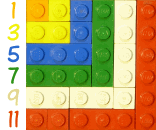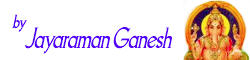or an Introduction to Pythagorean Triples first)

A "Pythagorean Triple" is a set of positive integers, a, b and c that fits the rule:

a2 + b2 = c2## Triangles

And when we make a triangle with sides a, b and c it will be a right angled triangle (see Pythagoras' Theorem for more details):Note:

• c is the longest side of the triangle, called the "hypotenuse"
• a and b are the other two sides

## Pythagorean Triples

A famous example of a Pythagorean Triples:32 + 42 = 52 9 + 16 = 25

Two more examples:5, 12, 13 9, 40, 41 52 + 122 = 132 92 + 402 = 412 25 + 144 = 169 (try it yourself)

## Endless

The set of Pythagorean Triples is endless.

We can prove this with the help of the first Pythagorean Triple (3, 4, and 5):

Let n be any integer greater than 1, then 3n, 4n and 5n are also a set of Pythagorean Triple. This is true because:

(3n)2 + (4n)2 = (5n)2

Examples:

n   (3n, 4n, 5n)
2   (6,8,10)
3   (9,12,15)
...   ... etc ...

So we can make infinitely many triples just using the (3,4,5) triple.

## Euclid's Proof that there are Infinitely Many Pythagorean Triples

But Euclid used a different reasoning to prove the set of Pythagorean Triples is unending.

The proof was based on the fact that the difference of the squares of any two consecutive (one after the other) numbers is always an odd number.

### Examples:

22 − 12 = 4 − 1 = 3 (an odd number),

32 − 22 = 9 − 4 = 5 (an odd number),

42 − 32 = 16 − 9 = 7 (an odd number),

etc

Just like this (can you see why?)Odd numbers and consecutive squares

See Squares and Odd Numbers, or have a look at this table as an example:

n n2 Difference
1 1
2 4 4−1 = 3
3 9 9−4 = 5
4 16 16−9 = 7
5 25 25−16 = 9
... ... ...

And there are an infinite number of odd numbers. Since the perfect squares form a subset of the odd numbers, and a fraction of infinity is also infinity, it follows that there must also be an infinite number of odd squares. So there are an infinite number of Pythagorean Triples.

## Properties

An interesting fact: a Pythagorean Triple always consists of:

• all even numbers, or
• two odd numbers and an even number.

A Pythagorean Triple can never be made up of all odd numbers or two even numbers and one odd number. This is true because:

• The square of an odd number is an odd number and the square of an even number is an even number.
• The sum of two even numbers is an even number and the sum of an odd number and an even number is in odd number.

So, when both a and b are even, c is even too. Similarly when one of a and b is odd and the other is even, c has to be odd!

## Constructing Pythagorean Triples

It is easy to construct sets of Pythagorean Triples.

When m and n are any two positive integers (m > n):

• a = m2 − n2
• b = 2mn
• c = m2 + n2

Then a, b, and c form a Pythagorean Triple.

### Example: m=2 and n=1

• a = 22 − 12 = 4 − 1 = 3
• b = 2 × 2 × 1 = 4
• c = 22 + 12 = 4 + 1 = 5

Thus, we obtain the first Pythagorean Triple (3,4,5).

Similarly, when m=3 and n=2 we get the next Pythagorean Triple (5,12,13).

## List of the First Few

Here is a list of all Pythagorean Triples where a, b, and c are less than 1,000.

But the list only has the first set (a,b,c) which is a Pythagorean Triple (called primitive Pythagorean Triples), so the multiples of (a,b,c), such as (2a,2b,2c), (3a,3b,3c), etc are not in the list.

Example: (3,4,5) is a Pythagorean Triple. (6,8,10) is also a Pythagorean Triple, but is not shown as it is just (3,4,5) times 2.

 (3,4,5) (5,12,13) (7,24,25) (8,15,17) (9,40,41) (11,60,61) (12,35,37) (13,84,85) (15,112,113) (16,63,65) (17,144,145) (19,180,181) (20,21,29) (20,99,101) (21,220,221) (23,264,265) (24,143,145) (25,312,313) (27,364,365) (28,45,53) (28,195,197) (29,420,421) (31,480,481) (32,255,257) (33,56,65) (33,544,545) (35,612,613) (36,77,85) (36,323,325) (37,684,685) (39,80,89) (39,760,761) (40,399,401) (41,840,841) (43,924,925) (44,117,125) (44,483,485) (48,55,73) (48,575,577) (51,140,149) (52,165,173) (52,675,677) (56,783,785) (57,176,185) (60,91,109) (60,221,229) (60,899,901) (65,72,97) (68,285,293) (69,260,269) (75,308,317) (76,357,365) (84,187,205) (84,437,445) (85,132,157) (87,416,425) (88,105,137) (92,525,533) (93,476,485) (95,168,193) (96,247,265) (100,621,629) (104,153,185) (105,208,233) (105,608,617) (108,725,733) (111,680,689) (115,252,277) (116,837,845) (119,120,169) (120,209,241) (120,391,409) (123,836,845) (124,957,965) (129,920,929) (132,475,493) (133,156,205) (135,352,377) (136,273,305) (140,171,221) (145,408,433) (152,345,377) (155,468,493) (156,667,685) (160,231,281) (161,240,289) (165,532,557) (168,425,457) (168,775,793) (175,288,337) (180,299,349) (184,513,545) (185,672,697) (189,340,389) (195,748,773) (200,609,641) (203,396,445) (204,253,325) (205,828,853) (207,224,305) (215,912,937) (216,713,745) (217,456,505) (220,459,509) (225,272,353) (228,325,397) (231,520,569) (232,825,857) (240,551,601) (248,945,977) (252,275,373) (259,660,709) (260,651,701) (261,380,461) (273,736,785) (276,493,565) (279,440,521) (280,351,449) (280,759,809) (287,816,865) (297,304,425) (300,589,661) (301,900,949) (308,435,533) (315,572,653) (319,360,481) (333,644,725) (336,377,505) (336,527,625) (341,420,541) (348,805,877) (364,627,725) (368,465,593) (369,800,881) (372,925,997) (385,552,673) (387,884,965) (396,403,565) (400,561,689) (407,624,745) (420,851,949) (429,460,629) (429,700,821) (432,665,793) (451,780,901) (455,528,697) (464,777,905) (468,595,757) (473,864,985) (481,600,769) (504,703,865) (533,756,925) (540,629,829) (555,572,797) (580,741,941) (615,728,953) (616,663,905) (696,697,985)